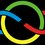# IMO Problems Weekly! (1990)The goal of this set of notes is to improve our problem solving and proof writing skills. You are encouraged to submit a solution to any of these problems, and join in the discussion in #imo-discussion. For more details, see IMO Problems Discussion Group.

So the wait for the IMO problems is over.....
To change from our rigorous yearly format, I have now switched to selected problems over the years! These are some really beautiful problems you should try your hand at! Post in your progress(even if it is little!) and we might find some out of the box Solutions! So here goes some problems from 1990:

Problem 1: Let $\mathbb{Q}^+$ be the set of positive rational numbers. Construct a function $f: \mathbb{Q}^+ \rightarrow \mathbb{Q}^+$ such that $f(xf(y))=\dfrac{f(x)}{y}$ , for all $x,y$ in $\mathbb{Q}^+$

Problem 2: Find all positive integers n having the property that $\dfrac{2^n +1}{n^2}$ is an integer.

Problem 3: Given a circle with two chords $AB,CD$ that meet at $E$, let $M$ be a point of chord $AB$ other than $E$. Draw the circle through $D, E$, and $M$. The tangent line to the circle $DEM$ at $E$ meets the lines $BC,AC$ at $F,G,$ respectively. Given $\dfrac{AM}{ AB} = \lambda$, ﬁnd $\dfrac{GE}{ EF}$ .

Problem 4: On a circle, $2n-1( n \geq 3)$ diﬀerent points are given. Find the minimal natural number $N$ with the property that whenever $N$ of the given points are colored black, there exist two black points such that the interior of one of the corresponding arcs contains exactly $n$ of the given $2n-1$ points.

###### This is part of the set International Mathematical OlympiadsNote by Sualeh Asif
5 years, 6 months ago

This discussion board is a place to discuss our Daily Challenges and the math and science related to those challenges. Explanations are more than just a solution — they should explain the steps and thinking strategies that you used to obtain the solution. Comments should further the discussion of math and science.

When posting on Brilliant:

• Use the emojis to react to an explanation, whether you're congratulating a job well done , or just really confused .
• Ask specific questions about the challenge or the steps in somebody's explanation. Well-posed questions can add a lot to the discussion, but posting "I don't understand!" doesn't help anyone.
• Try to contribute something new to the discussion, whether it is an extension, generalization or other idea related to the challenge.

MarkdownAppears as
*italics* or _italics_ italics
**bold** or __bold__ bold
- bulleted- list
• bulleted
• list
1. numbered2. list
1. numbered
2. list
Note: you must add a full line of space before and after lists for them to show up correctly
paragraph 1paragraph 2

paragraph 1

paragraph 2

[example link](https://brilliant.org)example link
> This is a quote
This is a quote
    # I indented these lines
# 4 spaces, and now they show
# up as a code block.

print "hello world"
# I indented these lines
# 4 spaces, and now they show
# up as a code block.

print "hello world"
MathAppears as
Remember to wrap math in $$ ... $$ or $ ... $ to ensure proper formatting.
2 \times 3 $2 \times 3$
2^{34} $2^{34}$
a_{i-1} $a_{i-1}$
\frac{2}{3} $\frac{2}{3}$
\sqrt{2} $\sqrt{2}$
\sum_{i=1}^3 $\sum_{i=1}^3$
\sin \theta $\sin \theta$
\boxed{123} $\boxed{123}$

Sort by:

Is the discussion on?

- 5 years, 3 months ago

May b off

- 5 years, 2 months ago

@Sharky Kesa @Xuming Liang @Abdur Rehman Zahid @Aareyan Manzoor
The next note in the series is up!
(I didn't forget to tag you this time Sharky)

- 5 years, 6 months ago